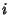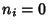## 15 PuzzleA puzzle introduced by Sam Loyd in 1878. It consists of 15 squares numbered from 1 to 15 which are placed in abox leaving one position out of the 16 empty. The goal is to rearrange the squares from a given arbitrary starting arrangement by sliding them one at a time into the configuration shown above. For some initial arrangements, this rearrangement is possible, but for others, it is not.

To address the solubility of a given initial arrangement, proceed as follows. If the Square containing the numberappears before'' (reading the squares in the box from left to right and top to bottom)numbers which are less than, then call it an inversion of order, and denote it. Then definewhere the sum need run only from 2 to 15 rather than 1 to 15 since there are no numbers less than 1 (somust equal 0). Ifis Even, the position is possible, otherwise it is not. This can be formally proved using Alternating Groups. For example, in the following arrangement(2 precedes 1) and all other, soand the puzzle cannot be solved.

References

Ball, W. W. R. and Coxeter, H. S. M. Mathematical Recreations and Essays, 13th ed. New York: Dover, pp. 312-316, 1987.

Bogomolny, A. Sam Loyd's Fifteen.'' http://www.cut-the-knot.com/pythagoras/fifteen.html.

Bogomolny, A. Sam Loyd's Fifteen [History].'' http://www.cut-the-knot.com/pythagoras/history15.html.

Johnson, W. W. Notes on the 15 Puzzle. I.''' Amer. J. Math. 2, 397-399, 1879.

Kasner, E. and Newman, J. R. Mathematics and the Imagination. Redmond, WA: Tempus Books, pp. 177-180, 1989.

Kraitchik, M. The 15 Puzzle.'' §12.2.1 in Mathematical Recreations. New York: W. W. Norton, pp. 302-308, 1942.

Story, W. E. Notes on the 15 Puzzle. II.''' Amer. J. Math. 2, 399-404, 1879.